# Calc

The program written in language Lisp2D, calculates forms set by strings. Parses the given expression for calculation and calculates it.
Calculator start:
> ./lisp2d calc.lisp2d

Accuracy of calculation is defined by possibilities of the interpreter - numbers are stored in a mode of fractions.
Binary functions are supported:
 + addition - subtraction * multiplication / division % remainder of division ^ degree
In the absence of argument of binary function the last result is meant. Unary operation a minus is not supported. It is necessary to write a negative number so:
-1 → 0-1
Functions evaluation is supported:
 abs absolute value |x| floor the integer part of number [x] floor(x,n) the integer part of division [x/n] ceil the minimum integer not the smaller x gcd(x,…,z) the greatest common diviser of integers lcm(x,…,z) the least common multiple of integers sqrt(x[,eps]) the square root √x [with supported quality eps] cbrt cube root exp exponent ex ln the log natural ln(x) log(base,x) the log with the base: logbase(x) ! factorial x! sin sine sin(x) cos cosine cos(x) tan tangent tan(x) cot cotangent cot(x) arcsin arcsine sin-1(x) arccos arccosine cos-1(x) arctan arctangent tan-1(x) arctan(y,x) arctangent from division tan-1(y/x) sinh sine hyperbolic sinh(x) cosh cosine hyperbolic cosh(x) tanh tangent hyperbolic tanh(x) cth cotangent hyperbolic cth(x) arsinh arcsine hyperbolic sinh-1(x) arcosh arccosine hyperbolic cosh-1(x) artanh arctangent hyperbolic tanh-1(x) degrees =x*180/π radians =x/180*π combin(x,n) =C(x,n)=n!/x!/(n-x)! combina(x,n) =n!/(n-x)! multinomial(x,…,z) =(x+…+z)!/x!…/z! erf(x) Gauss error function: 2/√π∫0x(e-t2)ⅆt ; 3 → 0.999978 inverf(x) 0.999978 → 3 num(x) numerator denom(x) denomirator round(x) rounding to integer value round(x,y) rounding in limits [x-y,x+y] real(x) real part of complex number imag(x) imagine part of complex number

There is a possibility to use mathematical constants pi and e.
Function without arguments random returns a random number [0…1).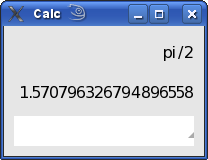Numbers, with the exponent are supported.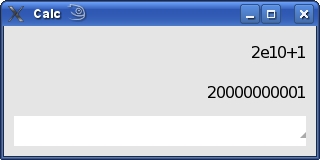It is authorised to use not parenthesis record for unary functions.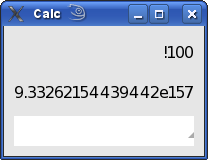Supports calculations set by means of brackets.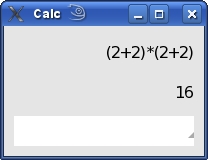For calculation it is possible to return the last task having pressed the button upwards.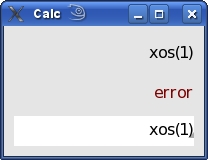Complex numbers? If you please…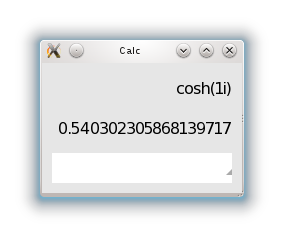For an exit it is necessary to press ESC or Ctrl+Q.
It is possible to start calculations not using a window.

> ./lisp2d calc.lisp2d "9^9" "x" "exp(2)"
387420489 error 7.38905609893